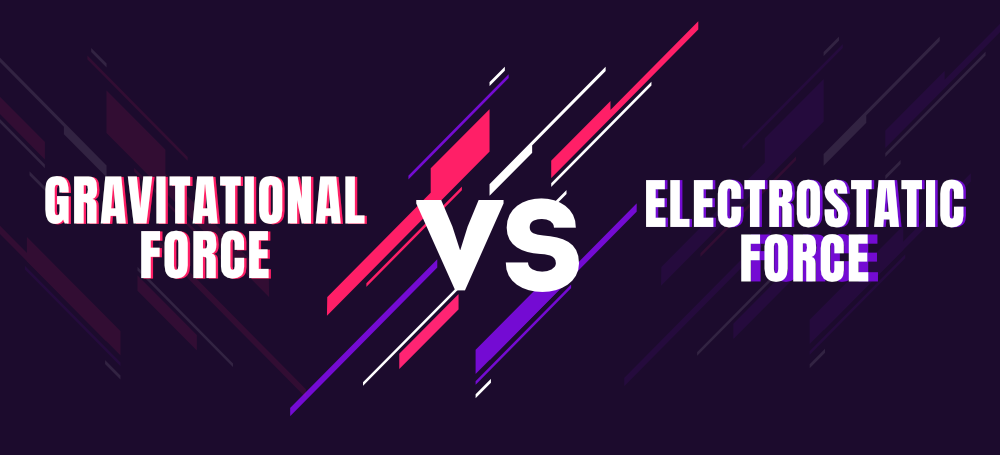# Difference between Gravitational Force and Electrostatic Force

• Last Updated : 30 Apr, 2022

Force is just defined as a push or pull of an object with the mass that causes it to change its velocity. It is an external agent that is capable of changing the state of a particular body neither in a rest position nor in motion. It has both magnitude and direction. It can be measured using a spring balance. The formula of force is F = ma, where m = mass of the object, a = acceleration.

Both Gravitational force and Electrostatic force are examples of Non-Contact forces. Non-Contact forces are defined as the force that acts through spaces without making any direct contact with the body.### Gravitational Force

Gravitational Force is defined as the force by which the earth attracts another object by mass. It is the force of attraction between all the masses in the universe, i.e. the attraction of the earth’s mass to bodies near its surface. Gravitational Force is the force that attracts any two objects with the mass

Formula of Gravitational Force

F = (G m1m2)/r2

Where,

G = Gravitational constant = 6.67 × 10-11 N. m2/kg2
m1 = mass of the object 1.
m2 = mass of the object 2.
r = distance between two objects.
S.I units = Newton (N).

### Electrostatic Force

It is defined as a force between objects due to the charge of the objects. It is the force exerted by a charged body on another charged body or an uncharged body. It is the force that comes when the bodies are not in contact. It is also defined as the attractive or repulsive force between two objects that are caused by their electric charge. It is also called Coulomb Force.

Formula of Electrostatic Force

F = (kq1q2)/r2

Where,

k = proportionality constant = 1/4π€o

Where €o = permittivity of a vacuum= 9 × 109 N.m2/c2.

q1 = charge of the object 1.

q2 = charge of the object 2.

r = distance between the two objects.

Difference between Gravitational Force and Electrostatic Force

### Sample Questions

Question 1: How much force is required to accelerate a 2000kg car at 5m/s2?

Given that,

mass of the car (m) = 2000kgs.

acceleration (a) = 5 m/s2.

F = ma

F = 2000 × 5

F = 10000 N.

Therefore, the force required is 10000 N.

Question 2: Two objects of mass 1000kg and 500 kg are kept at a distance of 400. Find the force of attraction between them

Given that,

m1= 1000kg

m2 = 500m

r = 400m

F = G m1m2/r2

= (6.67 × 10-11) × (1000 × 500)/(400)2

= (6.67 × 10-11) × 50/16

= 2.08 × 10-10 N.

Therefore, the force of attraction between them is 2.08 x 10-10 N.

Question 3: Two charges 3C and 6 C are kept at a distance of 9m away from each other. Find the force of attraction between them?

Given that,

q1= 3C

q2 = 6C

r = 9m

F = k.q1q2/r2

F = (9 × 109 × 3 × 6)/92

F= 2 × 109 N.

Therefore, the force of attraction between them is 2 × 109 N.

Question 4: Let us suppose 2 satellites are passing around the orbit, earth close to each other. For a moment they are part of each other if 400m. The masses of the satellite are 400kg and 1200kg. Calculate the gravitational force between them?

Given that,

m1 = 400kg

m2 = 1200kg

r = 400m

F = (6.67 × 10-11 × 400 × 1200)/(400)2

F = 20.01 × 10-11 N.

Therefore, the gravitational force between is 20.01 × 10-11N.

Question 5: A body of charges 10 C and 30C are kept at a distance of 10m away from each other. Find the electrostatic force between them?

Given that,

q1 = 10C

q2 = 30C

r = 10m

F = (9 × 109 × 10 × 30)/(10)2

F = 27 × 109 N.

Therefore, the electrostatic force between them is 27 × 109 N.

Question 6: An object having a mass of 2 kg is moving with a speed of 8 m/s hits a wall and comes to rest for 10sec. Calculate the force that makes the object stop.

Given that,

m = 2 kg

Initial velocity u = 8 m/s

Final velocity v = 0 m/s

Time = 10 sec.

Acceleration a = ( v – u)t

a = (0 – 8)10

a = 80

F = 2 × 80

F = 160 N.

Therefore, the force that is needed to stop the object = 160 N.

My Personal Notes arrow_drop_up